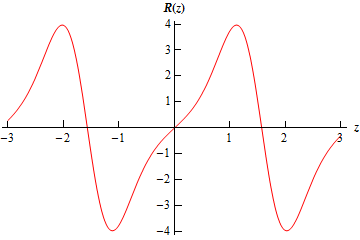Paul's Online Notes
Home / Calculus I / Applications of Integrals / Average Function Value
Show Mobile Notice Show All Notes Hide All Notes
Mobile Notice
You appear to be on a device with a "narrow" screen width (i.e. you are probably on a mobile phone). Due to the nature of the mathematics on this site it is best views in landscape mode. If your device is not in landscape mode many of the equations will run off the side of your device (should be able to scroll to see them) and some of the menu items will be cut off due to the narrow screen width.

### Section 6.1 : Average Function Value

The first application of integrals that we’ll take a look at is the average value of a function. The following fact tells us how to compute this.

#### Average Function Value

The average value of a continuous function $$f\left( x \right)$$ over the interval $$\left[ {a,b} \right]$$ is given by,

${f_{avg}} = \frac{1}{{b - a}}\int_{{\,a}}^{{\,b}}{{f\left( x \right)\,dx}}$

To see a justification of this formula see the Proof of Various Integral Properties section of the Extras chapter.

Let’s work a couple of quick examples.

Example 1 Determine the average value of each of the following functions on the given interval.
1. $$f\left( t \right) = {t^2} - 5t + 6\cos \left( {\pi \,t} \right)$$ on $$\left[ { - 1,\frac{5}{2}} \right]$$
2. $$R\left( z \right) = \sin \left( {2z} \right){{\bf{e}}^{1 - \cos \left( {2z} \right)}}$$ on $$\left[ { - \pi ,\pi } \right]$$
Show All Solutions Hide All Solutions
a $$f\left( t \right) = {t^2} - 5t + 6\cos \left( {\pi \,t} \right)$$ on $$\left[ { - 1,\frac{5}{2}} \right]$$ Show Solution

There’s really not a whole lot to do in this problem other than just use the formula.

\begin{align*}{f_{avg}} & = \frac{1}{{\frac{5}{2} - \left( { - 1} \right)}}\int_{{\, - 1}}^{{\,\frac{5}{2}}}{{{t^2} - 5t + 6\cos \left( {\pi \,t} \right)\,dt}}\\ & = \frac{2}{7}\left. {\left( {\frac{1}{3}{t^3} - \frac{5}{2}{t^2} + \frac{6}{\pi }\sin \left( {\pi t} \right)} \right)} \right|_{ - 1}^{\frac{5}{2}}\\ & = \frac{{12}}{{7\pi }} - \frac{{13}}{6}\\ & = - 1.620993\end{align*}

You caught the substitution needed for the third term right?

So, the average value of this function of the given interval is -1.620993.

b $$R\left( z \right) = \sin \left( {2z} \right){{\bf{e}}^{1 - \cos \left( {2z} \right)}}$$ on $$\left[ { - \pi ,\pi } \right]$$ Show Solution

Again, not much to do here other than use the formula. Note that the integral will need the following substitution.

$u = 1 - \cos \left( {2z} \right)$

Here is the average value of this function,

\begin{align*}{R_{avg}} & = \frac{1}{{\pi - \left( { - \pi } \right)}}\int_{{\, - \pi }}^{{\,\pi }}{{\sin \left( {2z} \right){{\bf{e}}^{1 - \cos \left( {2z} \right)}}\,dz}}\\ & = \left. {\frac{1}{{4\pi }}{{\bf{e}}^{1 - \cos \left( {2z} \right)}}} \right|_{ - \pi }^\pi \\ & = 0\end{align*}

So, in this case the average function value is zero. Do not get excited about getting zero here. It will happen on occasion. In fact, if you look at the graph of the function on this interval it’s not too hard to see that this is the correct answer.There is also a theorem that is related to the average function value.

#### The Mean Value Theorem for Integrals

If $$f\left( x \right)$$ is a continuous function on $$\left[ {a,b} \right]$$ then there is a number $$c$$ in $$\left[ {a,b} \right]$$ such that,

$\int_{{\,a}}^{{\,b}}{{f\left( x \right)\,dx}} = f\left( c \right)\left( {b - a} \right)$

Note that this is very similar to the Mean Value Theorem that we saw in the Derivatives Applications chapter. See the Proof of Various Integral Properties section of the Extras chapter for the proof.

Note that one way to think of this theorem is the following. First rewrite the result as,

$\frac{1}{{b - a}}\int_{{\,a}}^{{\,b}}{{f\left( x \right)\,dx}} = f\left( c \right)$

and from this we can see that this theorem is telling us that there is a number $$a < c < b$$ such that $${f_{avg}} = f\left( c \right)$$. Or, in other words, if $$f\left( x \right)$$ is a continuous function then somewhere in $$\left[ {a,b} \right]$$ the function will take on its average value.

Let’s take a quick look at an example using this theorem.

Example 2 Determine the number $$c$$ that satisfies the Mean Value Theorem for Integrals for the function $$f\left( x \right) = {x^2} + 3x + 2$$ on the interval $$\left[ {1,4} \right]$$.
Show Solution

First let’s notice that the function is a polynomial and so is continuous on the given interval. This means that we can use the Mean Value Theorem. So, let’s do that.

\begin{align*}\int_{{\,1}}^{{\,4}}{{{x^2} + 3x + 2\,dx}} & = \left( {{c^2} + 3c + 2} \right)\left( {4 - 1} \right)\\ & \left. {\left( {\frac{1}{3}{x^3} + \frac{3}{2}{x^2} + 2x} \right)} \right|_1^4 = 3\left( {{c^2} + 3c + 2} \right)\\ & \frac{{99}}{2} = 3{c^2} + 9c + 6\\ & 0 = 3{c^2} + 9c - \frac{{87}}{2}\end{align*}

This is a quadratic equation that we can solve. Using the quadratic formula we get the following two solutions,

\begin{align*}c & = \frac{{ - 3 + \sqrt {67} }}{2} = 2.593\\ c & = \frac{{ - 3 - \sqrt {67} }}{2} = - 5.593\end{align*}

Clearly the second number is not in the interval and so that isn’t the one that we’re after. The first however is in the interval and so that’s the number we want.

Note that it is possible for both numbers to be in the interval so don’t expect only one to be in the interval.